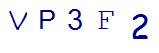# 4th Grade Math Help: 5 Ways to Conquer Factors and MultiplesLast Updated on August 3, 2023 by user

After kids learn multiplication, a typical 4th grade math curriculum then dives into factors and multiples.

Factors are what numbers can be multiplied together to so that they make another number (e.g., 1, 2, 3, and 6 are factors of 6). Multiples are what you get after multiplying a number by an integer (e.g., 20 is a multiple of 4).

Both are important to learn at this age because concepts such as least common multiples (LCM), greatest common factors (GCF), and equations with fractions are just around the corner.

If your child is starting to struggle when it comes to understanding factors and multiples, then it may be time to get them some fourth grade math help!

Here are 5 tricks you can try with your 4th grader if he or she needs to conquer factors and multiples:

## 1. Skip Counting

Skip counting is simply adding the same number over and over to get the desired result. This is a fundamental and foundational skill to have mastered to understand multiples.

For example, if you were multiplying 4×6, you might add 4+4+4+4+4+4 (that’s 6 times; kids might keep track on their fingers) to get the answer.

As you skip count, you also are identifying multiples – it’s an effective way to learn and remember them!

(Looking for some resources to practice skip counting? A math worksheet is a great way to provide practice! Thinkster Math‘s world-class curriculum includes Skip Counting. A Thinkster Math tutor can provide access to these math concepts so that your fourth grader get develop stronger proficiency in this fundamental skill.

## 2. Furious Factors

This is a math game you can play in the car when you are stuck in traffic.

It’s simple:

Give your child a two-digit number and ask him or her to list all the factors as quickly as possible. This might be a challenge without pencil and paper (and don’t expect perfection every time), but it does get kids thinking about factors on the spot. You can try this game at home by using two 10-sided dice to generate a number up to 100 from which to derive factors.

## 3. T-Charts

One method kids can use to visually figure out factors is a T-chart. Draw a capital T, and write the number above it. On the left side of the T will be the smaller factors; on the right will be the larger ones. Factor pairs will be directly across from each other. Let’s use 20 as an example. On the left side will be 1, 2, 4; on the right will be 20, 10, 5. Any factor that’s a square (such as 4 for 16) can be written on both sides.

## 4. The Rainbow Connection

Another way to derive factors is to create a rainbow chart.

Let’s go back to our example of 20. Write the factors across: 1, 2, 4, 5, 10, 20. Then draw a curved line from the 1 to the 20, from the 2 to the 10, and from the 4 to the 5. The result should look like a rainbow, and it’s a good way to remember that the larger and smaller the factors, the higher the line will climb.

Three good tips with this method: 1 will always be the first factor, the number itself will be the last factor, and if the number is even, 2 will be the second factor.

## 5. Factor Notebook

Challenge your fourth grade student to use either T-charts or rainbows to create a factor notebook. Instruct him or her to figure out the factors for every number to 100. This will be less of a cheat sheet (after all, your kids still will need to derive factors on the fly) and more of a reference guide that will come in handy for 4th grade math help as well as for concepts, such as GCFs and prime numbers, that they will eventually be taught.

## Still having trouble with fourth grade math concepts? Hire an online math tutor!

Divisibility rules driving your child crazy? (We have some math hacks for those!)

Or, is it geometric figures, multi digit dividends, place value understanding, or unit fractions?Fourth grade math can be challenging for many students. The curriculum takes all basic math skills learned to this point and requires mastery in them as shifting to higher-level concepts. (Hello, tricky word problem!). Crucial skills are developed, as your child continues to build the skills needed for pre-Algebra and Algebra.

If your child continues to struggle, hiring a math tutor is a great option. An elite Thinkster Math tutor can work with your fourth grader and provide daily support and guidance for your child to make incredible learning improvements.SummaryArticle Name
4th Grade Math Help: 5 Ways to Conquer Factors and Multiples
Description
Is your child struggling with factors and multiples? Here's how you can provide 4th grade math help to improve these concepts.
Author
Publisher Name
Thinkster Math
Publisher Logo

## Subscribe to Thinkster Blog## Recommended Articles### Unlocking the Code: Teaching Mathematical Symbols and Their Meaning

Knowledge of mathematical symbols is a foundational skill that empowers students to engage with and excel i...